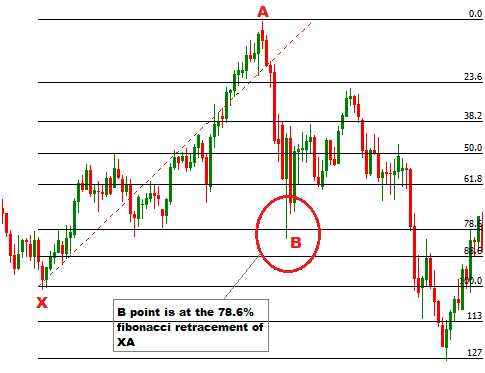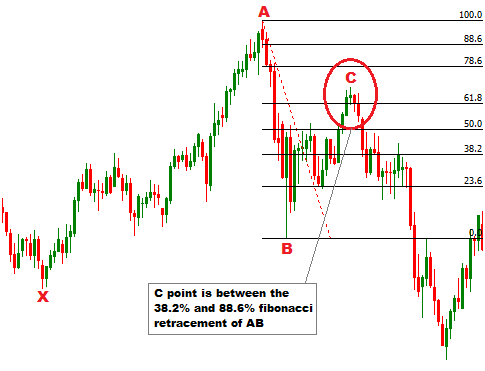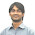Welcome! Dear Traders,you are reading my forex trading experiences. Forex trading is a very profitable and very risky business opportunity. If you are a beginner, calm down,have a cup of coffee, and convince yourself that you need to study hard to win in forex trading. Obviously, the task is not easy as the statistics claim that only 5% traders win in forex trading. If you are determined, serious,and hard working, you can surely be included in the group of winners.

HOW TO TRADE BULLISH BUTTERFLY HARMONIC CHART PATTERN

Bullish butterfly harmonic chart pattern provides a short entry signal when it is completed, or when the D point of the pattern is confirmed. This post explains the way to find or draw a bullish butterfly harmonic chart pattern, and the way to trade a bullish butterfly harmonic chart pattern.

How to find or draw a bullish butterfly harmonic chart pattern on price chart?
To draw a bullish butterfly harmonic chart pattern, first of all, we are to find the X and A points of the pattern. Then we have to find the B point of the pattern. To find the B point of the pattern, we have to draw a fibonacci retracement tool from X to A. The B point should be at the 78.6% fibonacci retracement of XA.

To find the B point without fibonacci retracement tool, we will use the following formulas-

(IA) AB= (price at X point-price at A point) X 0.786
(IB) Price level at 78.6% of XA= (result found from formula (IA) + price at point A)

The B point of this pattern should lie on the price levels found from formula (IB). This satisfies the AB/XA=0.786 condition of the pattern. Look at the following illustration.Next, we will draw a fibonacci retrcement tool from A to B to find the C point of the pattern. C point should be between the 38.2% and 88.6% fibonacci retracement of AB.

To find the C point without fibonacci retracement tool, we will use the following formulas-

(IA) BC= (price at B point-price at A point) X 0.382
(IB) Price level at 38.2% of AB= (price at point B-result found from formula (IA))
(IIA) BC= (price at B point-price at A point) X 0.886
(IIB) Price level at 88.6% of AB= (price at point B-result found from formula (IIA))

The C point of this pattern should lie between the price levels found from formula (IB) and (IIB). This satisfies the BC/AB=0.382-0.886 condition of the pattern. Look at the following illustration.In the next step, we will draw a fibonacci retracement tool from B to C to confirm the D point of the pattern. The D point should be between the 161.8% and 224% fibonacci retracement of BC.

To find the D point without fibonacci retracement tool, we will use the formulas-

(IA) CD= (price at B point-price at C point) X 1.618
(IB) Price level at 161.8% of AB= (price at point C+result found from formula (IA))
(IIA) CD= (price at B point-price at C point) X 2.24
(IIB) Price level at 224% of AB= (price at point C+result found from formula (IIA))

The D point of this pattern should lie between the price levels found from formula (IB) and (IIB). This satisfies the CD/BC=1.618-2.24 condition of the pattern. Look at the following illustration.We know that D point is the entry point of the pattern, that is why, D point is reconfirmed. To reconfirm the D point of the pattern, we will draw a fibonacci retracement from X to A. D point should be between the 127% and 141% fibonacci retracement of XA.

To find the D point without fibonacci retracement tool, we will use the formulas-

(IA) AD= (price at X point-price at A point) X 1.27
(IB) Price level at 127% of XA= (price at point A+result found from formula (IA))
(IIA) CD= (price at B point-price at C point) X 1.41
(IIB) Price level at 141% of AB= (price at point C+result found from formula (IIA))

In this case we can see, accurately satisfied the AD/XA= 1.27-1.41 condition of the pattern. See the illustration below.

Remember, the D point is the entry point of the pattern, and it is confirmed by two conditions. A reliable D point should satisfy the both conditions, but it is also accepted, if the one condition is satisfied.

How to trade bearish butterfly harmonic chart pattern?
A short entry is suggested when the D point of the pattern in confirmed. The stop loss for the order should be placed at the upper resistance level. The take profit for the order should be placed at the 38.2-88.6% fibonacci retracement of CD, depending on the fundamental analysis.

B and C points can also be traded, if the entries are confirmed by other technical analysis tool(s).

Thank You and Good Luck.

1.It will not be more easy if we use zigzag indicators together to find the butterfly harmonic?

1.I don't think zigzag indicator is a tool to draw a harmonic chart pattern.

2.3.2.